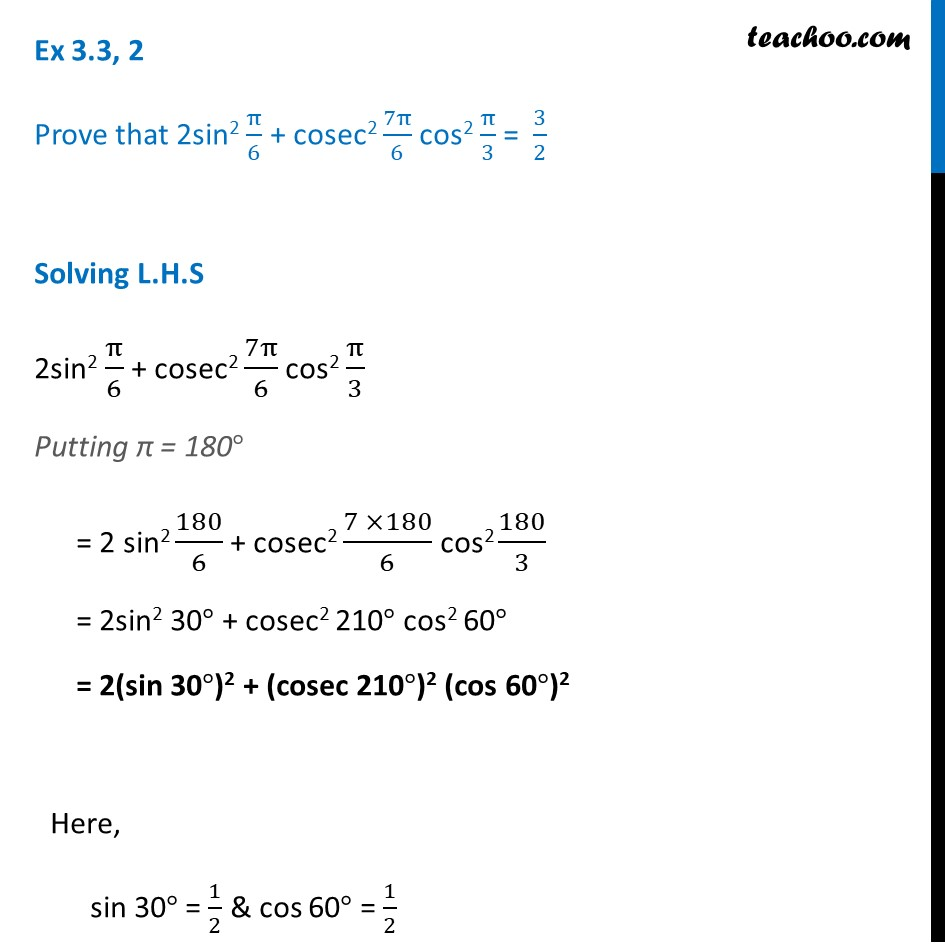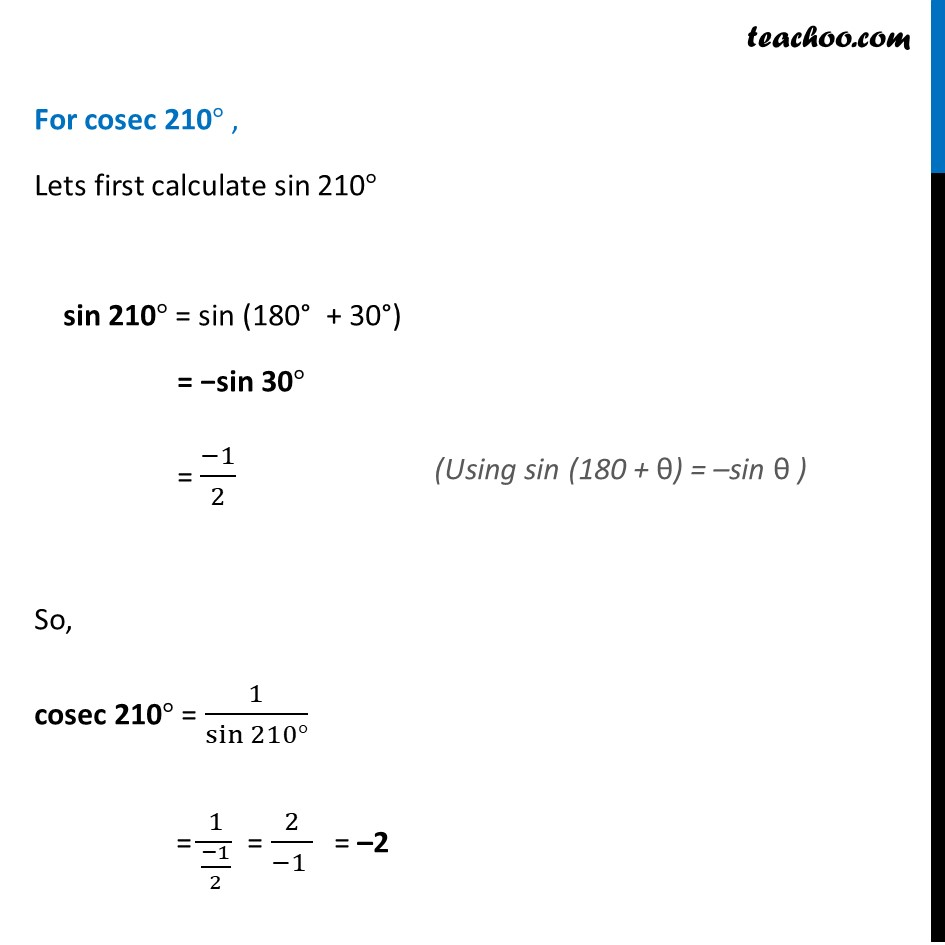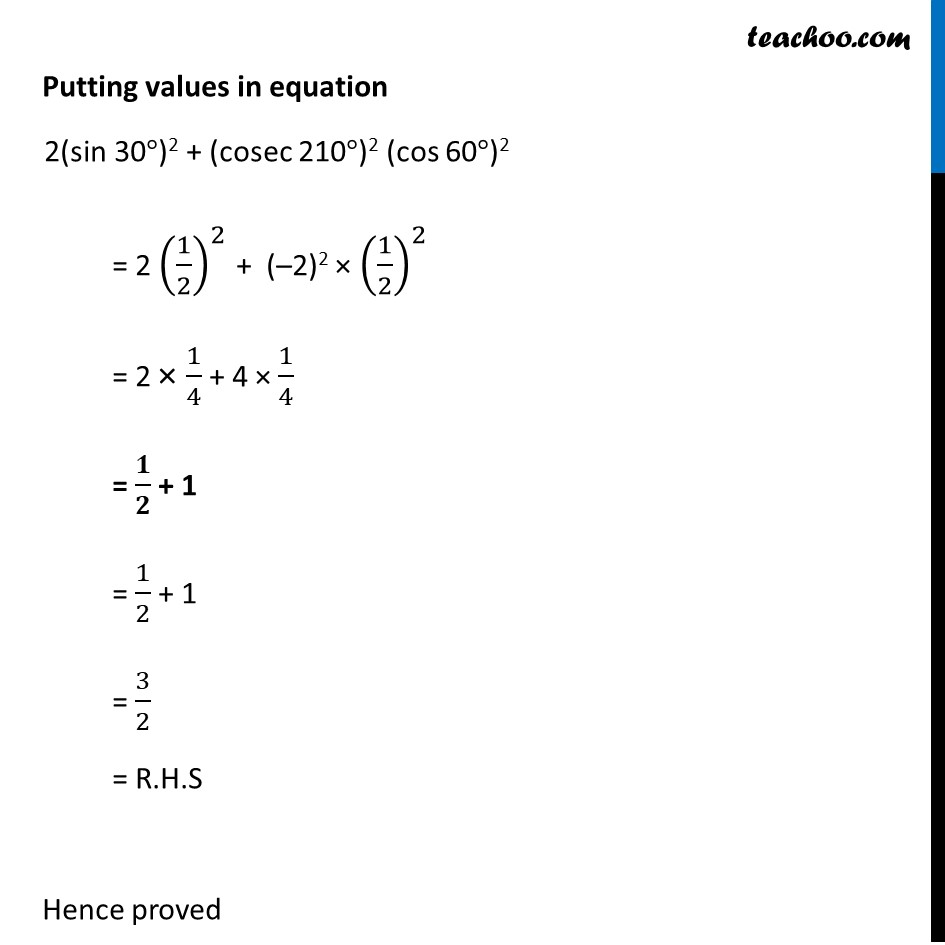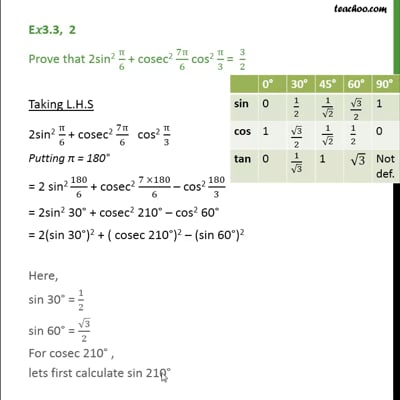Ex 3.3

Chapter 3 Class 11 Trigonometric Functions
Serial order wiseThere is some mistake in this Video. Please check images above.This video is only available for Teachoo black users

Learn in your speed, with individual attention - Teachoo Maths 1-on-1 Class

### Transcript

Ex 3.3, 2 Prove that 2sin2 π/6 + cosec2 7π/6 cos2 π/3 = 3/2 Solving L.H.S 2sin2 π/6 + cosec2 7π/6 cos2 π/3 Putting π = 180° = 2 sin2 180/6 + cosec2 (7 ×180)/6 cos2 180/3 = 2sin2 30° + cosec2 210° cos2 60° = 2(sin 30°)2 + (cosec 210°)2 (cos 60°)2 For cosec 210° , Lets first calculate sin 210° sin 210° = sin (180° + 30°) = −sin 30° = (−1)/2 So, cosec 210° = 1/sin⁡〖210°〗 = 1/((−1)/2) = 2/(−1 ) = –2 Putting values in equation 2(sin 30°)2 + (cosec 210°)2 (cos 60°)2 = 2 (1/2)^2 + (–2)2 × (1/2)^2 = 2 × 1/4 + 4 × 1/4 = 𝟏/𝟐 + 1 = 1/2 + 1 = 3/2 = R.H.S Hence proved